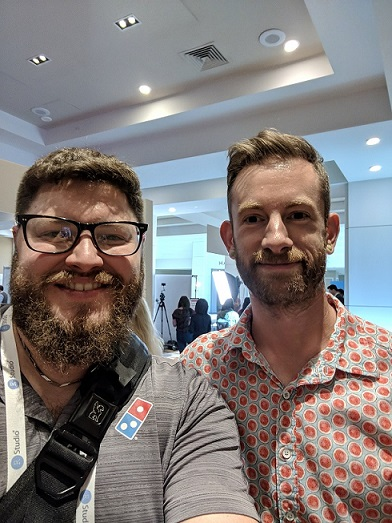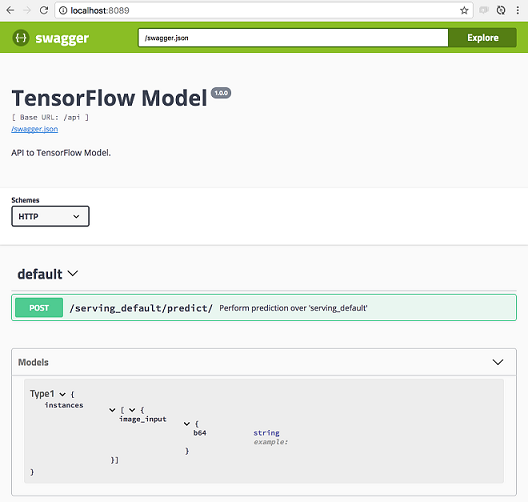February 8, 2018

## Introduction

• data scientist @dominos
• R evangelist
• recent attendee of rstudio::conf
• "… I guess if you use ifelse() you're doing AI" - JJ Allaire

Production and Interoperability

• database best practices
• building APIs
• TensorFlow API

## Highlight## Database best practices

• tl;dr don't use RODBC
• use odbc + DBI instead
# connect using locally-defined Domain Name System (DNS)
conn <- DBI::dbConnect(odbc::odbc(), "MyDataMart")
# connect using DB-specific driver
conn <- DBI::dbConnect(
odbc::odbc(),
driver = "SQL Server",
server = "Server",
database = "DB",
port = 12345
)

## Database best practices

query <- "
SELECT
flight
,tailnum
,origin
FROM flights
ORDER BY origin
"

dataset <- DBI::dbGetQuery(conn, query)

message <- DBI::dbWriteTable(conn, "iris", iris)

## Database best practices

• using dplyr functions
flights_db <- tbl(conn, "flights")

# set up the query
tailnum_delay_db <- flights_db %>%
group_by(tailnum) %>%
summarise(
delay = mean(arr_delay),
n = n()
) %>%
arrange(desc(delay)) %>%
filter(n > 100)

# show the query
tailnum_delay_db %>% show_query()

# execute query and collect the data
tailnum_delay <- tailnum_delay_db %>% collect()

## Database best practices

• using pool to manage DB connections
• mostly relevant for Shiny developers
• handles in-app connections (active and idle)
• one/app –> fast but no simultaneous requests
• one/query –> slow but allows simultaneous requests
pool <- pool::dbPool(
odbc::odbc(),
driver = "SQL Server",
server = "Server",
database = "DB",
port = 12345
)

dataset <- pool %>%
tbl("flights") %>%
collect()

## Building APIs

# myfile.R

#* @get /mean
normalMean <- function(samples=10){
data <- rnorm(samples)
mean(data)
}

#* @post /sum
as.numeric(a) + as.numeric(b)
}
library(plumber)
r <- plumb("myfile.R")
r\$run(port=8000)

## R interface to Keras

• all the functionality of Python interface
• achieved through reticulate
# instantiate the model
model <- application_resnet50(weights = 'imagenet')

img_path <- "images/elephant.jpg"
img <- image_load(img_path, target_size = c(224,224))
x <- image_to_array(img)

# preprocess the input for prediction using resnet50
x <- array_reshape(x, c(1, dim(x)))
x <- imagenet_preprocess_input(x)

# make predictions then decode and print them
preds <- model %>% predict(x)
imagenet_decode_predictions(preds, top = 3)[]

## Deploying TF models

• train and export models from keras
• test local deployment with tfdeploy
• deploy into production with cloudml and/or rsconnect (not shown)
# Run local server with model
tfdeploy::serve_savedmodel("count-pepperoni")

## Deploying TF models## Bonus: embedding TF models in JavaScript!

• package kerasjs converts model to JS
# Install kerasjs from GitHub
devtools::install_github("rstudio/kerasjs")

# Train and Export model from Keras as HDF5
# or use an existing model
model_path <- system.file(
"models/keras-mnist.hdf5",
package = "kerasjs"
)

# Convert model to JavaScript and Preview
kerasjs_convert(model_path)